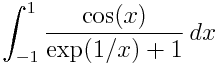# Integration trick

Here’s a clever example from Paul Nahin’s new book Inside Interesting Integrals. Suppose you want to evaluateSince the range of integration is symmetric around zero, you might think to see whether the integrand is an odd function, in which case the integral would be zero. (More on such symmetry tricks here.) Unfortunately, the integrand is not odd, so that trick doesn’t work directly. However, it does help indirectly.

You can split any function f(x) into its even and odd parts.The integral of a function over a symmetric interval is the integral of its even part because its odd part integrates to zero. The even part of the integrand above works out to be simply cos(x)/2 and so the integral evaluates to sin(1).

## 6 thoughts on “Integration trick”

1. GlennF

Neat trick. Using the same basic trick it follows that for any even function g_e(x) and any odd function g_o(x) that:
$\int_{-1}^{1} \frac{g_e(x)}{\exp(g_o(x)) + 1} \, d x = \frac{1}{2} \int_{-1}^{1} g_e(x) \,d x$

(Which looks hard to believe).

2. Marco Valli

Hi John. I had already spotted that book on Amazon! Sounds interesting…is it worth buying?! Best, Marco

3. Yes, I’d say it’s worth buying.

4. Hey John,

Thanks for mentioning “Inside Interesting Integrals”… Bought it for Christmas. :)

Do you know any book like that about differential equations?

5. Thanks. Unfortunately I don’t know of such a book for differential equations. If you write one, let me know. :)

6. Inside Interesting Integrals is definitely worth buying.